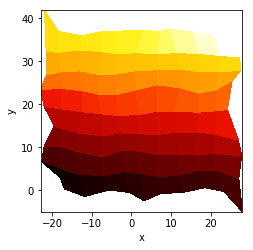Title
Dependencies
Matplotlib
Backends
Matplotlib
Bokeh
In :
import numpy as np
import holoviews as hv
hv.extension('matplotlib')A QuadMesh represents 2D rectangular grid expressed as x- and y-coordinates defined as 1D or 2D arrays. Unlike the Image type, a QuadMesh may be regularly or irregularly spaced and contain either bin edges or bin centers. If bin edges are supplied, the shape of the x/y-coordinate arrays should be one greater than the value array shape.

The default interface expects data to be specified in the form:

QuadMesh((X, Y, Z))



where X and Y may be 1D or 2D arrays of the shape N(+1) and M(+1) respectively or N(+1)xM(+1) and the Z value array should be of shape NxM. Other gridded formats such as xarray are also supported if installed.

The grid orientation follows the standard matrix convention: An array Z with shape (nrows, ncolumns) is plotted with the column number as X and the row number as Y. See the Gridded Datasets user guide for all the other accepted data formats.

Here is a simple QuadMesh with logarithmic sampling along the 'x' dimensions:

In :
n = 8  # Number of bins in each direction
xs = np.logspace(1, 3, n)
ys = np.linspace(1, 10, n)
zs = np.arange((n-1)**2).reshape(n-1, n-1)
print('Shape of x-coordinates:', xs.shape)
print('Shape of y-coordinates:', ys.shape)
print('Shape of value array:', zs.shape)

Shape of x-coordinates: (8,)
Shape of y-coordinates: (8,)
Shape of value array: (7, 7)

Out: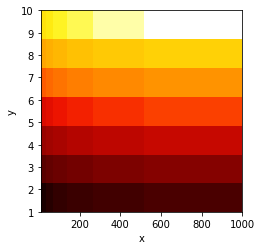The coordinate system of a QuadMesh is defined by the bin edges, therefore any index falling into a binned region will return the appropriate value. As the bin edges have continuous values, you can use non-linear axes such as log axes:

In :
qmesh = hv.QuadMesh((xs, ys, zs), label='Linear Axis')
log_qmesh = qmesh.relabel('Log x-axis')
qmesh + log_qmesh.opts(logx=True)

Out: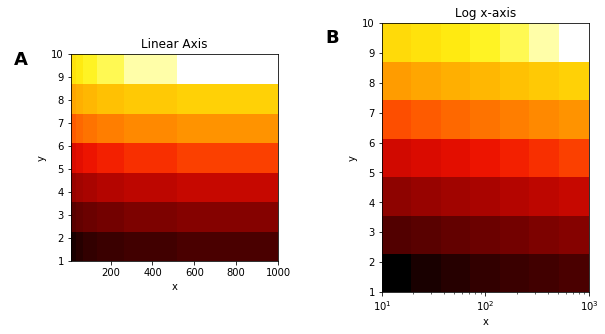Unlike Image objects, slices must be inclusive of the bin edges but otherwise the slicing semantics are the same. The reason for this difference is that QuadMesh is really a two-dimensional histogram and when slicing, you only want to see the bins that fall within the specified slice ranges.

In the next example, we specify a slice along the x- and y-axis to extract the lower corner and we set the z-dimension range to maintain the full color range of the colormap:

In :
qmesh = hv.QuadMesh((xs, ys, zs))
qmesh[20:400, :8].redim.range(z=qmesh.range('z'))

Out: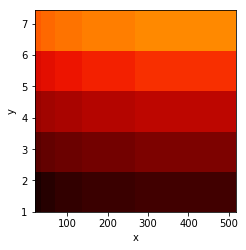To use an interactive hover tool to inspect the sample values, you can use QuadMesh with the hover tool in the Bokeh backend.

For full documentation and the available style and plot options, use hv.help(hv.QuadMesh).

## Irregular meshes¶

In addition to axis aligned meshes like those we worked with above, a QuadMesh may also be used to represent irregular or unstructured meshes. In this example we will create an irregular mesh consisting of 2D X, Y and Z arrays defining the position and value of each simplex in the mesh:

In :
n=20
coords = np.linspace(-1.5,1.5,n)
X,Y = np.meshgrid(coords, coords);
Qx = np.cos(Y) - np.cos(X)
Qy = np.sin(Y) + np.sin(X)
Z = np.sqrt(X**2 + Y**2)

print('Shape of x-coordinates:', Qx.shape)
print('Shape of y-coordinates:', Qy.shape)
print('Shape of value array:', Z.shape)

qmesh

Shape of x-coordinates: (20, 20)
Shape of y-coordinates: (20, 20)
Shape of value array: (20, 20)

Out: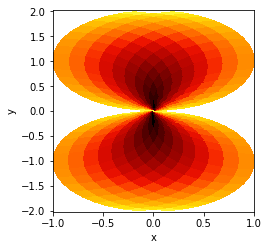To illustrate irregular meshes a bit further we will randomly jitter the mesh coordinates along both dimensions, demonstrating that QuadMesh may be used to represent completely arbitrary meshes. It may also be used to represent overlapping meshes, however the behavior during slicing and other operations may not be well defined in such cases.

In :
np.random.seed(13)
xs, ys = np.meshgrid(np.linspace(-20, 20, 10), np.linspace(0, 30, 8))
xs += xs/10 + np.random.rand(*xs.shape)*4
ys += ys/10 + np.random.rand(*ys.shape)*4

zs = np.arange(80).reshape(8, 10)